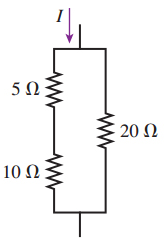# Problem: The 10Ω resistor in the figure (Figure 1) is dissipating 20 W of power.How much power is the 5Ω resistor dissipating?How much power is the 20Ω resistor dissipating?

###### FREE Expert Solution
83% (40 ratings)View Complete Written Solution
###### Problem Details

The 10Ω resistor in the figure (Figure 1) is dissipating 20 W of power.

How much power is the 5Ω resistor dissipating?

How much power is the 20Ω resistor dissipating?Frequently Asked Questions

What scientific concept do you need to know in order to solve this problem?

Our tutors have indicated that to solve this problem you will need to apply the Solving Resistor Circuits concept. You can view video lessons to learn Solving Resistor Circuits. Or if you need more Solving Resistor Circuits practice, you can also practice Solving Resistor Circuits practice problems.

What professor is this problem relevant for?

Based on our data, we think this problem is relevant for Professor Yao's class at GSU.